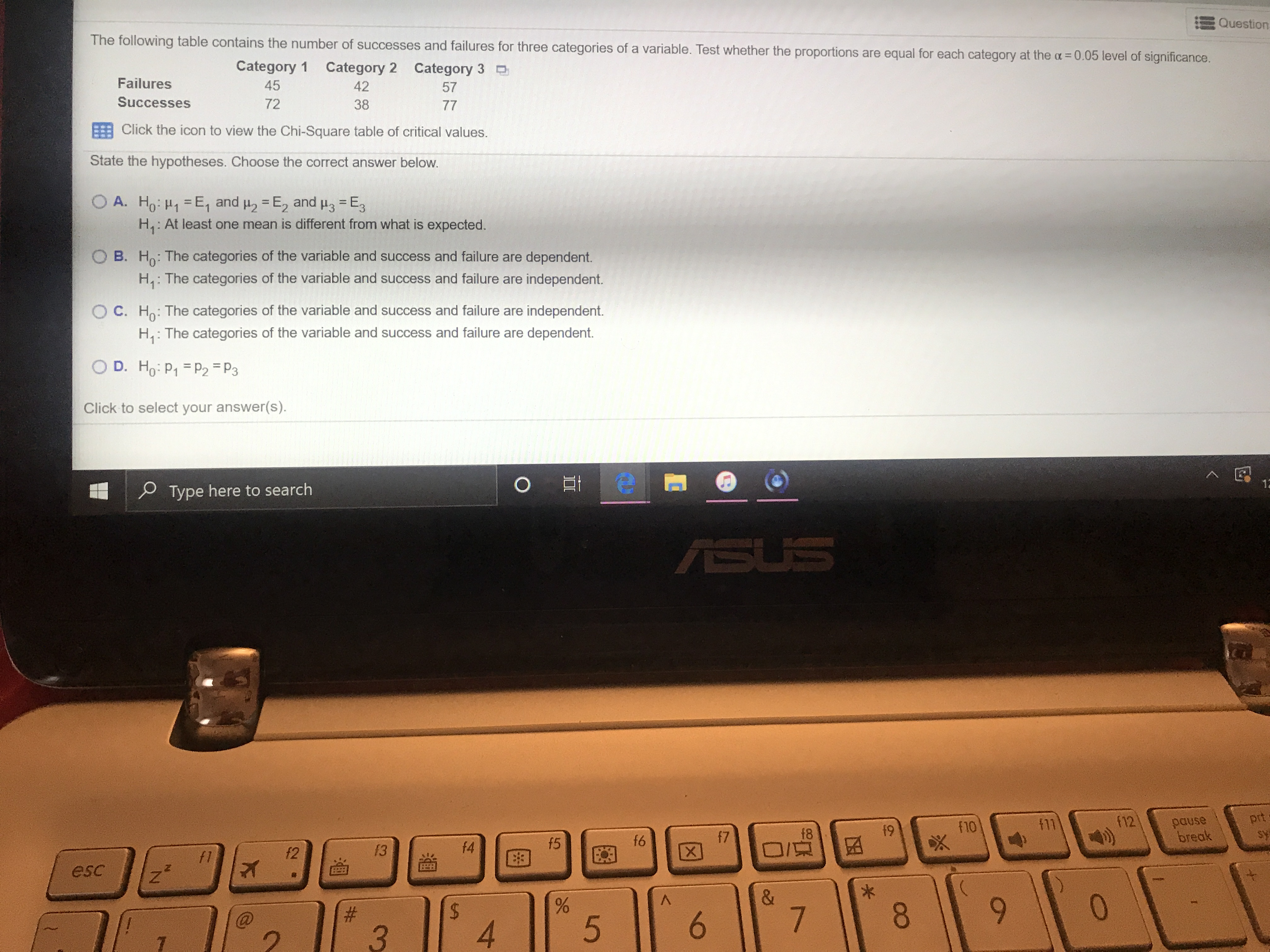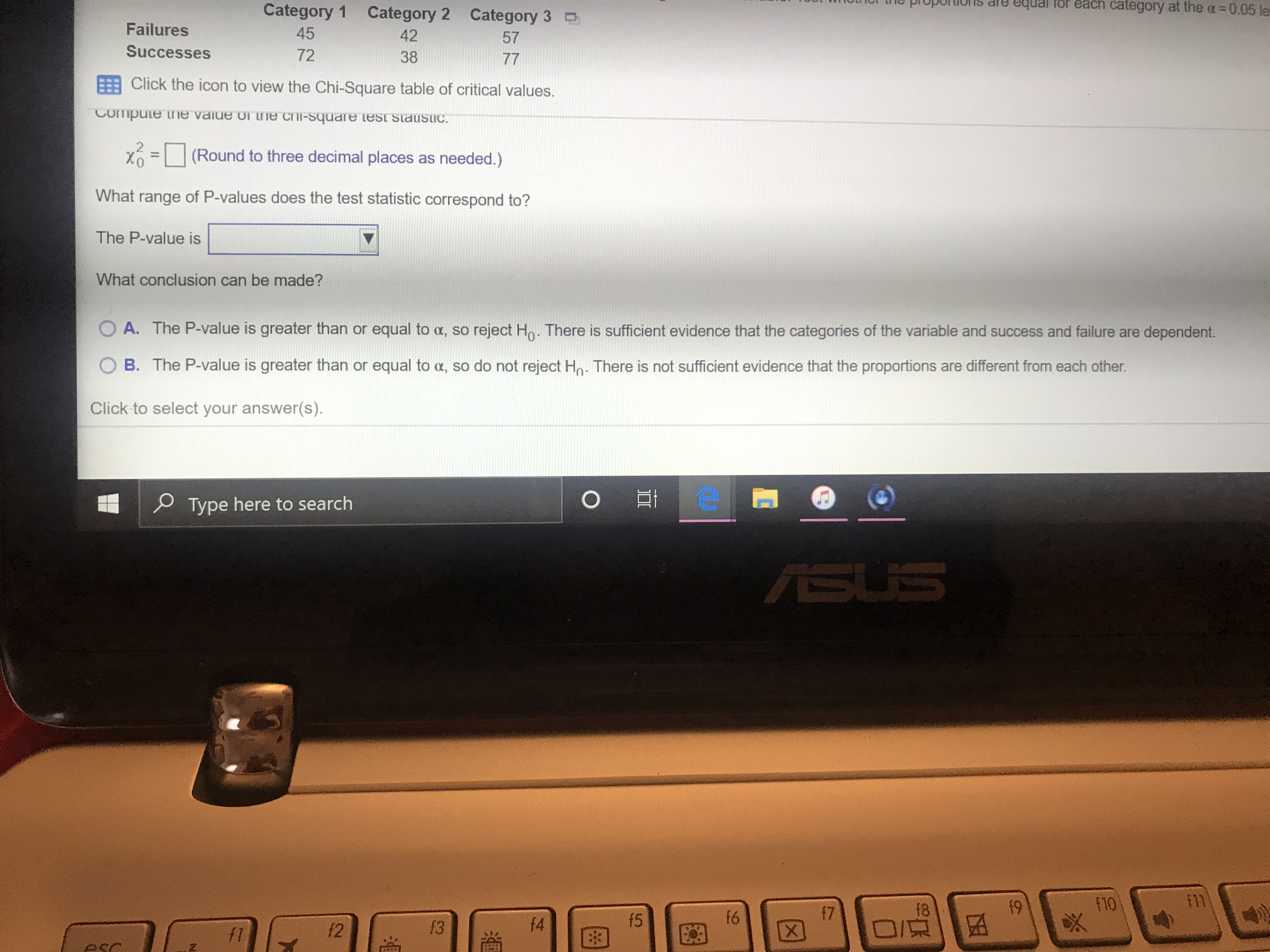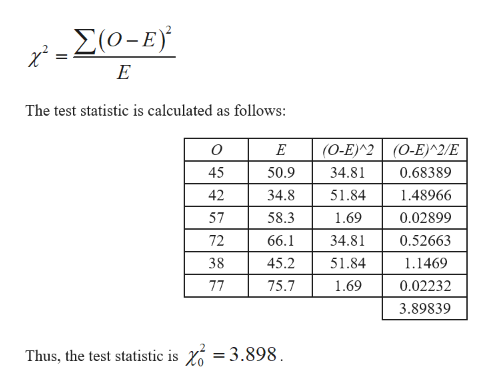# %233.QuestionThe following table contains the number of successes and failures for three categories of a variable. Test whether the proportions are equal for each category at the a =D0.05 level of significance.Category 1Category 2 Category 3 DFailures454257Successes723877Click the icon to view the Chi-Square table of critical values.State the hypotheses. Choose the correct answer below.A. Ho: H1 =E, and u, = E, and u3 = E,H3 = E3H,: At least one mean is different from what is expected.B. Ho: The categories of the variable and success and failure are dependent.H,: The categories of the variable and success and failure are independent.C. Ho: The categories of the variable and success and failure are independent.H,: The categories of the variable and success and failure are dependent.D. Ho: P1 =P2 =P3Click to select your answer(s).P Type here to search12ASUSf11(12prtf10pausebreakf5f6f7f8f9f1f213f4esc%2446.7.8.6. TUUL WIIOTCI tne propUIIons areequal for each category at the a=0.05leCategory 1 Category 2 Category 3 DFailures454257Successes723877Click the icon to view the Chi-Square table of critical values.Compute ne value oI Lhe chi-square test slaustic.X =(Round to three decimal places as needed.)What range of P-values does the test statistic correspond to?The P-value isWhat conclusion can be made?O A. The P-value is greater than or equal to a, so reject Ho. There is sufficient evidence that the categories of the variable and success and failure are dependent.B. The P-value is greater than or equal to a, so do not reject Ho. There is not sufficient evidence that the proportions are different from each other.Click to select your answer(s).P Type here to searchASUSf8f9f10f11f5f6f7f2f3f4f1E3

Question
2 views

Helphelp_outlineImage Transcriptionclose%23 3. Question The following table contains the number of successes and failures for three categories of a variable. Test whether the proportions are equal for each category at the a =D0.05 level of significance. Category 1 Category 2 Category 3 D Failures 45 42 57 Successes 72 38 77 Click the icon to view the Chi-Square table of critical values. State the hypotheses. Choose the correct answer below. A. Ho: H1 =E, and u, = E, and u3 = E, H3 = E3 H,: At least one mean is different from what is expected. B. Ho: The categories of the variable and success and failure are dependent. H,: The categories of the variable and success and failure are independent. C. Ho: The categories of the variable and success and failure are independent. H,: The categories of the variable and success and failure are dependent. D. Ho: P1 =P2 =P3 Click to select your answer(s). P Type here to search 12 ASUS f11 (12 prt f10 pause break f5 f6 f7 f8 f9 f1 f2 13 f4 esc %24 4 6. 7. 8. 6. fullscreenhelp_outlineImage TranscriptioncloseTUUL WIIOTCI tne propUIIons are equal for each category at the a=0.05le Category 1 Category 2 Category 3 D Failures 45 42 57 Successes 72 38 77 Click the icon to view the Chi-Square table of critical values. Compute ne value oI Lhe chi-square test slaustic. X = (Round to three decimal places as needed.) What range of P-values does the test statistic correspond to? The P-value is What conclusion can be made? O A. The P-value is greater than or equal to a, so reject Ho. There is sufficient evidence that the categories of the variable and success and failure are dependent. B. The P-value is greater than or equal to a, so do not reject Ho. There is not sufficient evidence that the proportions are different from each other. Click to select your answer(s). P Type here to search ASUS f8 f9 f10 f11 f5 f6 f7 f2 f3 f4 f1 E3 fullscreen
check_circle

Step 1

The hypotheses are given below:

H0: The categories of the variable and the successes and failures are independent.

H1: The categories of the variable and the success and failures are dependent.

Thus, the correct answer is option (C).

Step 2

Test Statistic:

Before calculating the test statistic find the expected frequency for each cell,

Expected frequency:

Step 3

Similarly for the remaining cells, the expected frequencies are calculated and the values...help_outlineImage Transcriptionclose- Σ(ο-E) The test statistic is calculated as follows: (O-E)^2 (0-E)^2/E 45 50.9 34.81 0.68389 51.84 42 34.8 1.48966 57 58.3 1.69 0.02899 72 66.1 34.81 0.52663 45.2 38 51.84 1.1469 77 75.7 1.69 0.02232 3.89839 Thus, the test statistic is Y = 3.898. fullscreen

### Want to see the full answer?

See Solution

#### Want to see this answer and more?

Solutions are written by subject experts who are available 24/7. Questions are typically answered within 1 hour.*

See Solution
*Response times may vary by subject and question.
Tagged in

### Other